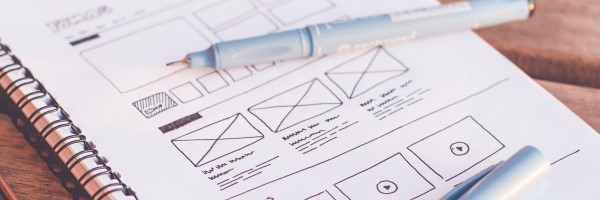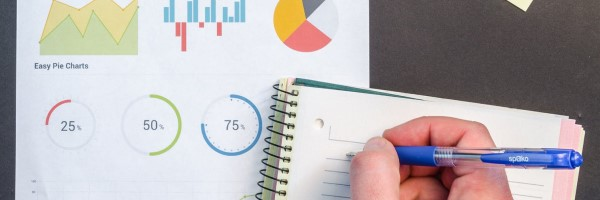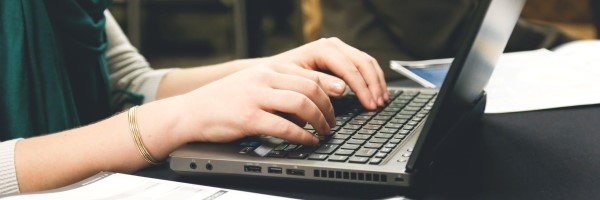Final Exam Information

• The STAT 107 Final Exam has two parts: a CBTF-based exam and a Python notebook.
• Infinite Practice Exams Available on PrairieLearn
• You must sign up for your CBTF exam on the CBTF scheduler. Choose anytime you want to take it between Thursday, May 2 - Thursday, May 9!
• The Python exam will be available on Compass 2g starting on Friday, May 3.
• Both parts combined are designed to take no more than 3 hours.

May. 1, 2019Lecture 38-39: k-means Clustering

Apr. 29, 2019Lecture 37: Distance Metrics and Clustering

In many areas of Data Science, we need to define how different two rows of data are from each other. The most common way to find this difference is to define a distance metric that can be used to provide a numeric difference or "distance" two rows of data are from each other.

Apr. 24, 2019Lab 10: Hypothesis Tests

Apr. 23, 2019Lecture 36: A/B Testing

With hypothesis testing in hand, we can explore how you can go about creating experiments in the real-world that allow you to testing of hypotheses and adding value to a project! One of the most common techniques in the use of A/B Testing.

Apr. 22, 2019Lecture 35: t Test

T-tests are very similar to z-tests. They test if a difference we observe is due to chance. We use t-tests and the Student's Curve (t-Distribution) only when a set of ALL THREE conditions are met.

Apr. 17, 2019Lab 10: Lists

Apr. 16, 2019Lecture 34: The Two Sample Z Test

Previously, we tested hypotheses about population averages (means) or percentages using the test statistic. Now we’ll try to test hypotheses that compare the averages (means) or percentages in two populations.

Apr. 15, 2019Lecture 33: Z Tests in Python

Apr. 12, 2019Lab 9: CLT

Apr. 9, 2019Lecture 32: One Sample Z Test

Hypothesis Tests are statistical tests to see if a difference we observe is due to chance. Many times, we have competing hypotheses about the value of a population parameter. It’s impossible or impractical to examine the whole population to find out which hypothesis is true, so we take a random sample and see which hypothesis better supported by our sample data..

Apr. 8, 2019Lecture 30+31: Sampling, Expected Value, and Standard Error

In our discussion of random variables, we started with games of chance because they easily translate into probability models. We know all of the outcomes and their probabilities. In the next section, we are going to see how random variables relate to gathering information about large populations from small samples.

Apr. 3, 2019Lab 9: Normal

Apr. 2, 2019Lecture 28-29: The Normal Distribution

Many histograms are close to the normal curve. For these histograms, you can use the normal curve to estimate percentages for the data.

Mar. 27, 2019Lecture 26: Random Variables

Statisticians use the term random variable for variables whose numeric values are based on the outcome of a random process. The domain of a random variable is the set of possible outcomes. Each outcome has a probability associated with it.

Mar. 27, 2019Lab 8: Loops

Mar. 26, 2019Lecture 25: Control Flow in Python - Simulation

Mar. 15, 2019Lecture 24: Control Flow in Python - Loops and Functions

In nearly every programming language, every program runs from top-to-bottom, one line at a time. In addition to running from top-to-bottom, there are three control flow commands in Python that allows us to control the flow of a Python program.

Mar. 15, 2019Lecture 23: Control Flow in Python - Conditionals and Loops

In nearly every programming language, every program runs from top-to-bottom, one line at a time. In addition to running from top-to-bottom, there are three control flow commands in Python that allows us to control the flow of a Python program.

Mar. 13, 2019Lab 7: Birthday

Mar. 12, 2019Lecture 22: Simulation and Analysis

Mar. 11, 2019Lecture 21: Binary Event Simulation

As we work towards simulating events using Python, we need to first develop an understanding of different types of events to simulate. The first type of events are events with exactly two outcomes, or binary outcome events.

Mar. 8, 2019Lecture 20: Simulation

Simulation is an imitation of a real-world event within a computer program. We can use millions of simulations and observe the distribution of outcomes to help us understand the answer to a problem that may be difficult to model mathematically.

Mar. 6, 2019Lecture 18+19: Probability

Feb. 27, 2019Lab 6: Regression

Feb. 26, 2019Lecture 17: Descriptive Statistics and Probability

Feb. 25, 2019Lecture 16: Correlation and Regression

Feb. 22, 2019Lecture 15: Correlation and Regression

Feb. 20, 2019Lab 5: Plots

Feb. 19, 2019Lecture 14: Scatter Plots

Just like histograms, box plots are used as a way to visually represent numerical data. They do this through selected percentiles which are given special names.

Feb. 15, 2019Lecture 13: Boxplots

Just like histograms, box plots are used as a way to visually represent numerical data. They do this through selected percentiles which are given special names.

Feb. 15, 2019Lecture 12: Center and Spread

Parameters are numerical facts about the population. In this lecture, we will look at parameters such as the average (µ) and standard deviation (σ) of a list of numbers. Later, we will start talking about statistics. Statistics are estimates of parameters computed from a sample.

Feb. 13, 2019Project 1: Confounding Variables among Excellent Teachers

Feb. 12, 2019Lecture 11: Bar Graphs and Histograms

Large tables of numbers can be difficult to interpret, no matter how organized they are. Sometimes it is much easier to interpret graphs than numbers.

Feb. 11, 2019Lecture 10: Data Cleaning and Review

Feb. 8, 2019Lecture 9: Functions and Data Cleaning

Feb. 6, 2019Homework 2: Simpson's Paradox

Homework 2 is available, due Sunday, Feb. 10 at 11:59pm

Feb. 5, 2019Lab 4: Similarity

Find your Data Science DISCOVERY twin using Data Science!

Feb. 5, 2019Lecture 8: Developing Algorithms for Complex Problems

Feb. 4, 2019Lecture 7: Creating Columns and Groups

Feb. 1, 2019Lab 3: Experimental Design

Begin to create your own control and treatment groups with while doing some experimental design!

Jan. 29, 2019Lecture 6: Introduction to Pandas

Time to focus in on data, learning the primary tool we will be using all semester!

Jan. 28, 2019Homework 1: Experimental Design and Privacy

Homework 1 is available, due Sunday, Feb. 3 at 11:59pm

Jan. 27, 2019Lecture 5: Data Science Tools

"Data", "Science", and "Tools" all have meaning in their own, explore how one relates to another and how they all related to Data Science DISCOVERY!

Jan. 25, 2019Lecture 4: Observational Studies & Simpson’s Paradox

For years observational studies have shown that people who carry lighters are more likely to get lung cancer. However, this does not mean that carrying lighters causes you to get cancer. Smoking is an obvious confounder! If we weren’t sure about this, how can we determine whether it’s the lighters or the confounders or (maybe some combination of both) that is causing the lung cancer?

Jan. 23, 2019Lab 2: Introduction to Pandas

Use `pandas` for the first time and read/process CSV files!

Jan. 22, 2019Lecture 3: Observational Studies & Confounders

Observational studies are done out of necessity. Whenever possible, it’s better to do a randomized controlled experiment. Why?

Jan. 16, 2019Lecture: Ideal Experimental Design

Does the death penalty have a deterrent effect? Is chocolate good for you? What causes breast cancer? All of these questions attempt to assign a cause to an effect. A careful examination of data can help shed light on questions like these.

Jan. 16, 2019Lab: Introduction to Data Science

In lab this week, you will get set up with the tools of Data Science (python, jupyter, git, and others) as well as complete your first notebook!

Jan. 15, 2019Welcome to Data Science Discovery

First lecture is Monday, Jan. 14 at 9am in G32 FLB. See you there!

Jan. 14, 2019